# 10 Easy Math Tricks That Could Really Make You Smarter

Image Credit: iStock

I know that math is some people’s things, but it’s definitely not mine – and I know that I’m not alone. Give me words any day of the week, y’all.

Of course, none of us are spared from needing to do math in real life. I’m sorry to say that the teachers were right about that.

If you’re like me and you need a little help, you’re not going to believe how easy these 10 tricks really are.

# 10. Dividing by 10, 100, or 1000.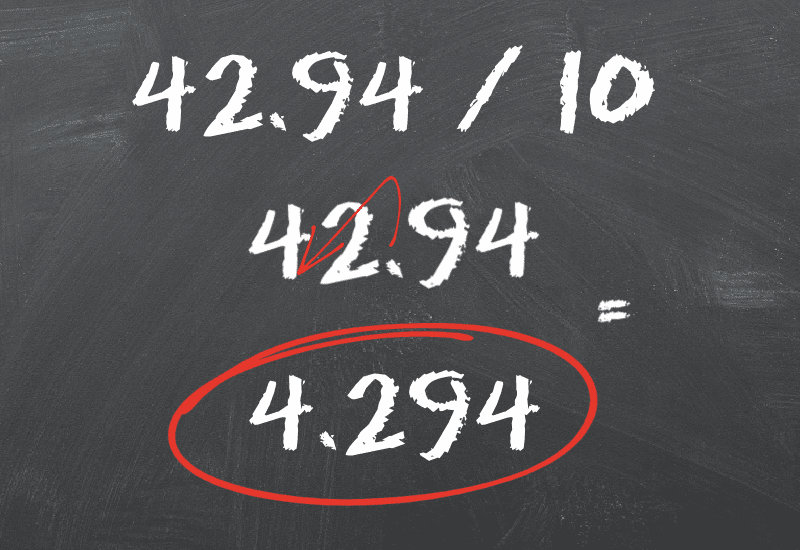To divide any number by 10, all you have to do is move the decimal place one spot to the left of the original number.

For diving by 100, that’s two spots, and for 1,000, three spots.

Example: 42.94/10 = 4.294.

# 9. How to easily calculate a 20% tip.

Kate Snow, author of The Math Facts That Stick, says tipping should be easy – if you want to leave 20% (and you leave at least 20%, right??), all you have to do is whip out your phone and divide the bill by 5.

And then round up. Just do it.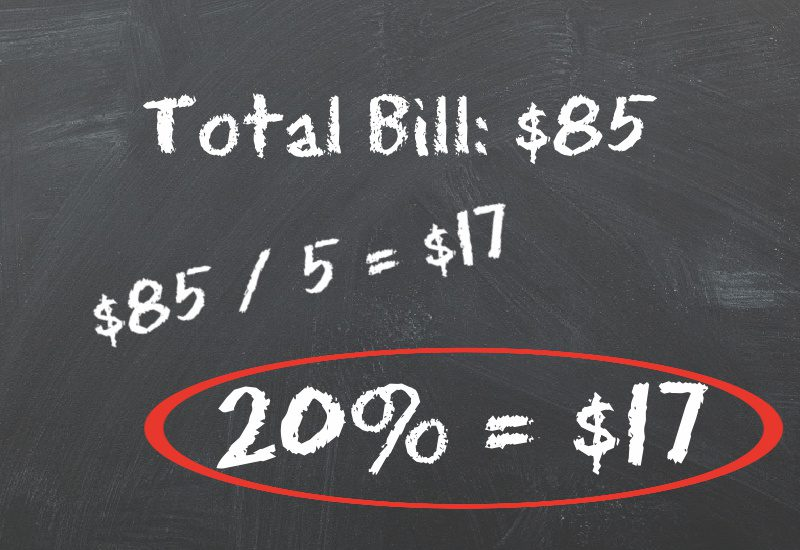# 8. Multiplying by 10, 100, or 1000.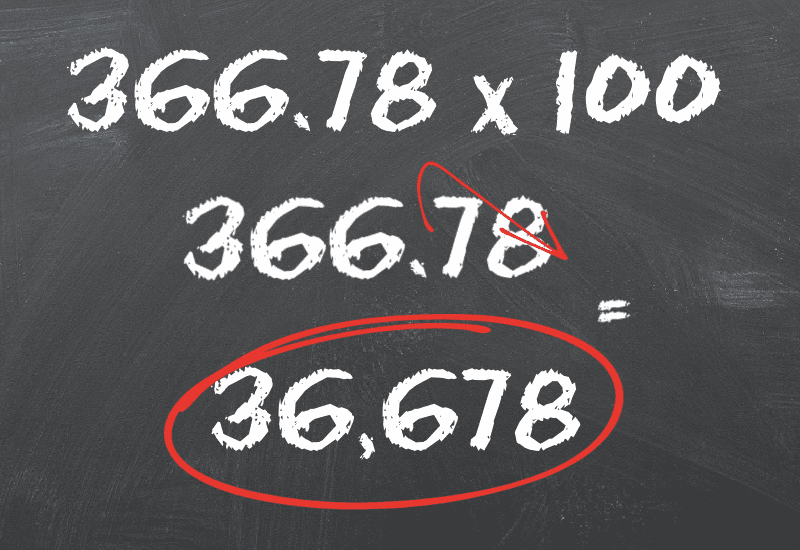Remember how we divided by these numbers by moving the decimal 1, 2, or 3 spots to the left?

If you’re multiplying, you move the decimal one place to the right – 1, 2, or 3 spots.

Example: 366.78 x 100 = 36,678.

# 7. Easily multiply 2-digit numbers by 11.This clever trick comes from math.hmc.edu, and involves simply adding the two digits together, then placing the sum between the original two numbers.

Example: 25 x 11 = (2+5=7) 275.

# 6. Turn a repeating decimal into a fraction.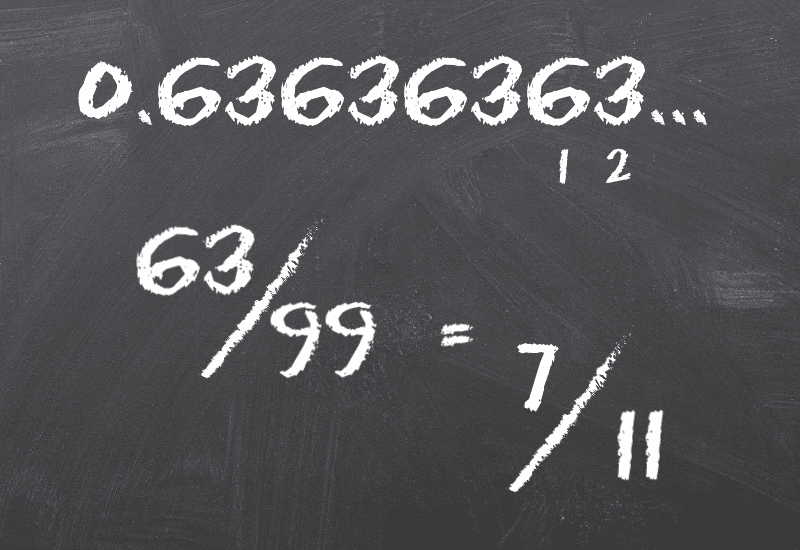You know those annoying decimals that just keep going and going and going forever? If you want to turn them into something easier to manage, like a fraction, use this tip from businessinsider.com.

You find the repeating number, then note the number of places it has. Then divide the repeating number by the a number of 9s with the same amount of places.

Example: 0.63636363 = (63/99) 7/11.

# 5. To double a large number.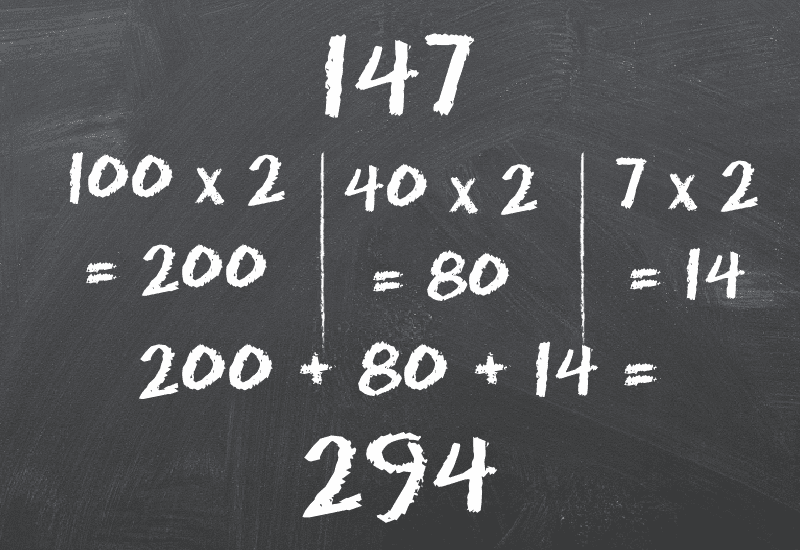Here, Snow says the easiest thing to do is to multiply each individual number by two, then add them together.

She also suggests starting from the left so you don’t lose track.

“For example, to double 147, start with the hundreds place. Double 100 is 200. Double 40 is 80. Double seven is 14. Add them all up (200 plus 80 plus 14) and you get 294.”

# 4. Multiply by 25.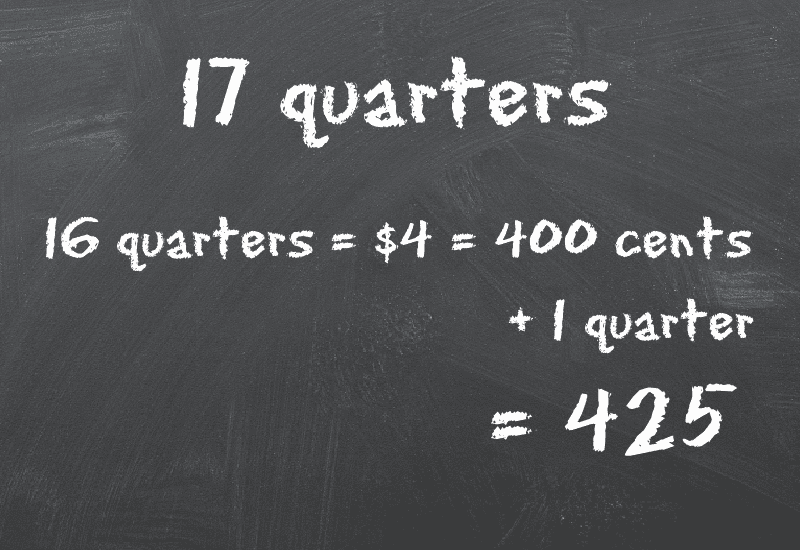Think of 25s by quarters, and Snow says everything will seem easier in your mind.

“If you had 17 quarters, how much money would you have? Every four quarters make a dollar, so 16 of the quarters equals four dollars or 400 cents. The extra quarter adds 25 cents, for a total of 425 cents.”

# 3. Multiply numbers that end in 0.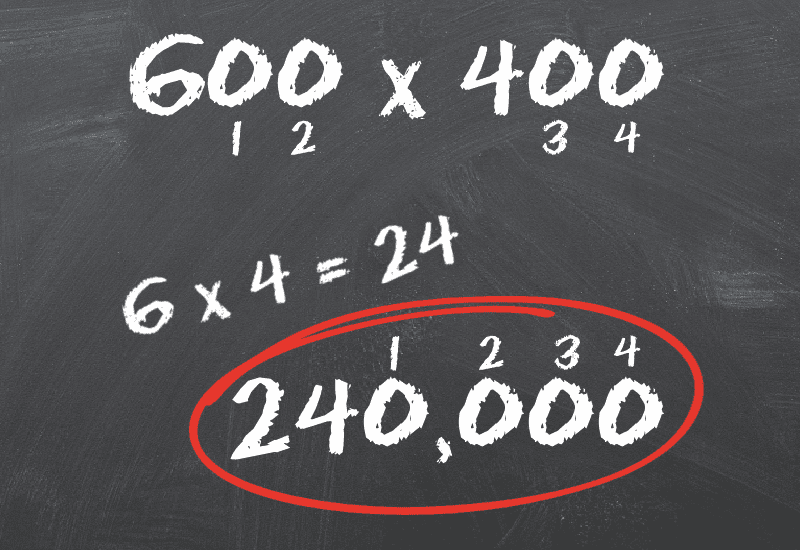No matter how large a number is sitting in front of you, if it ends in 0, you can solve it easily with this handy trick from University of Portland.

Just delete the zeros from the equation, then add them back in when you’re done.

Example: 600 x 400 = (6 x 4 = 24) 240,000.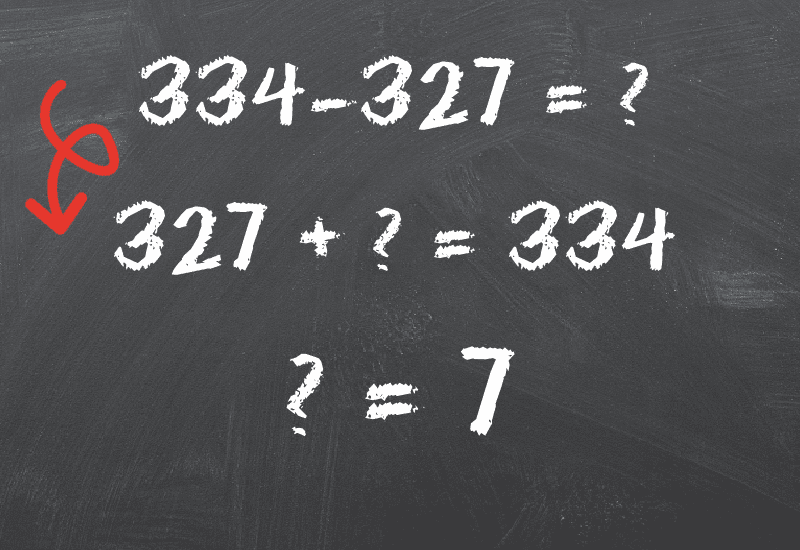Adding is easier than subtracting for many people, so just switch the two around in your head if that works better for you, says Snow.

“Instead of trying to take away 327 from 334, think of it as ‘327 plus how many more equals 334?’”

# 1. Multiplying by 9.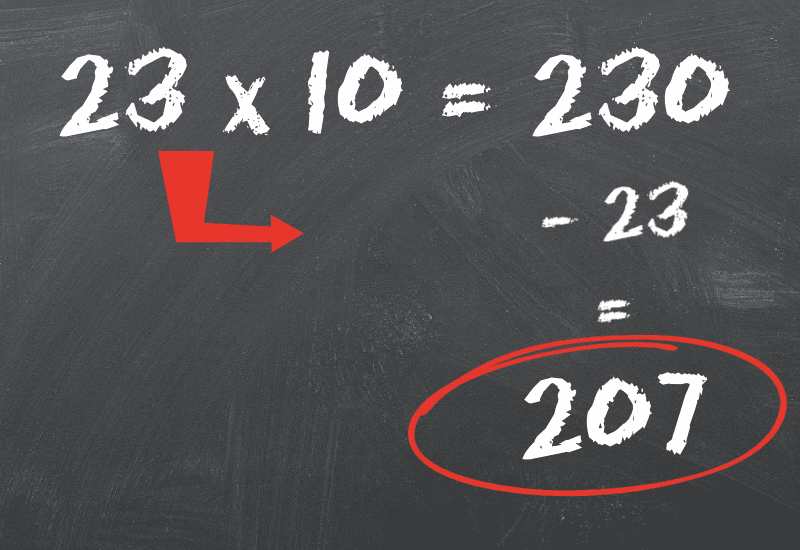Snow says that 9 is one of the times tables you don’t really need to memorize-  you just multiply by 10 first, then subtract the original number.

Example: 23 x 9 = (23 x 10 = 230) (230-23) 207.

Are you blown away? Because I kind of am.

What’s the best math tip you know that more people should? Share it with us in the comments!